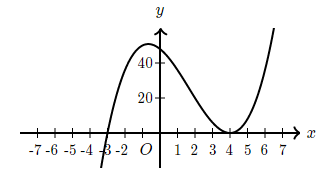mycollegehive
Which of the following could be the equation of the graph a...
SAT-math-test SAT math0Which of the following could be the equation of the graph above?

A. y = 2(x - 3)(x + 4)

B. y = (x - 2)(x + 3)(x - 4)

C. y = 2(x + 3)(x - 4)

D. y = (x + 3)(x - 4)2

459 viewsShareFollowUniversity of Benin Nigeria
19 November 2020School not set Nigeria
19 November 20200y = (x + 3)(x - 4)2Share

### Groups

How to insert math formulas/equations### Related Tags

SAT-math-test

14 followers

476 questionsSAT

9 followers

487 questionsmath

23 followers

1262 questions